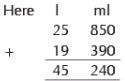Courses

# Olympiad Test: Measurement - 1

## 10 Questions MCQ Test Mathematics Olympiad Class 4 | Olympiad Test: Measurement - 1

Description
This mock test of Olympiad Test: Measurement - 1 for Class 4 helps you for every Class 4 entrance exam. This contains 10 Multiple Choice Questions for Class 4 Olympiad Test: Measurement - 1 (mcq) to study with solutions a complete question bank. The solved questions answers in this Olympiad Test: Measurement - 1 quiz give you a good mix of easy questions and tough questions. Class 4 students definitely take this Olympiad Test: Measurement - 1 exercise for a better result in the exam. You can find other Olympiad Test: Measurement - 1 extra questions, long questions & short questions for Class 4 on EduRev as well by searching above.
QUESTION: 1

### Shraddha has written following statements about the metric unit she would use to measure some objects. Find the incorrect sentence among them: 1. Centimetre is used to measure the length of a pencil. 2. Kilometre is used to measure distance from any city to another. 3. Metre is used to measure depth of a bucket. 4. Metre is used to measure height of a tree.

Solution:

Statement 3 is incorrect.

QUESTION: 2

Solution:
QUESTION: 3

### If 1 metre : 100 centimetre : : then 1 kilometre : ?

Solution:

We know 1 kilometre = 1000 metre.

QUESTION: 4

25l 850 ml and 19l 390 ml.

Solution:QUESTION: 5

17l 708 ml and 13l 993 ml

Solution:
QUESTION: 6

75l 95 ml and 12l 938 ml

Solution:
QUESTION: 7

A container has 2550 ml of water. How many litres and millilitres of water is in the container?

Solution:

We have 2550 ml = (2000 + 550) ml
= 2000 ml + 550 ml
= 2l 550 ml

QUESTION: 8

Rohan bought a 50 lt container of oil. He used 44 litres 300 ml of it. How much oil is left?

Solution:
QUESTION: 9

A jar can hold 4l 250 ml honey. How much honey will be needed to fill 4 jars?

Solution:

Required money = 4 × (4l + 250) ml
= 16l + 1000 ml
= 16l + 1l
= 17l

QUESTION: 10

Shraddha needs 6 containers which can hold 15l 600 ml oil. Find the capacity of each container.

Solution: